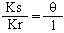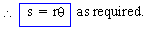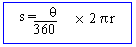Support the Monkey! Tell All your Friends and TeachersHome MonkeyNotes Printable Notes Digital Library Study Guides Study Smart Parents Tips College Planning Test Prep Fun Zone Help / FAQ How to Cite New Title Request

1.2 Arc Length & Area of The Circle

Arc lengthO is the centre of the circle of radius 'r' (r > 0) . A central angle of qc radians intercepts an arc AB of length 's'. We know that angle subtended at the centre (is proportional to the ) length of the arc.

\ qc = K . s .....(1)

where K is a constant.
Let `C' be any point on this circle such that Ð BOC = 1 radian so that length of arc BC = r

\ 1c = K . r .....(2)

Comparing the two relations (1) and (2) we have =Your browser does not support the IFRAME tag.

Note : In this formula q is always in radians with r and s are measured in the same units of length.

But when qis measured in degrees,For military purpose this written as W = Rm where m is the central angle in mils. R is the radius (range) expressed in thousands of meters and W is the chord (width) expressed in meters.

Index